>>

Login or Register   Integers on a Number Line
 New User Register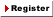Login Member: Email: Password:
 Site Search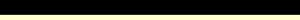Advance Search

Other animationsIntegers - Arithmetic Operations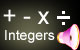Animation product List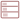Row formatGrid format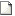List format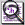Overview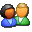(For age - group : Below 11 )

Fully animated ‘Integers on Number Line ’ covers the concept using real life examples. This will help you understand the rules of number line and how integers and dates are placed on it.Product - Animation
 Size/Duration Subscribe Preview / Trailer Size (KB) 10159 US\$    1.50 Rs.118.50  Check price in your currency Subscription Days = 30 Watch a preview (opens in separate window) Duration (hr:min:sec) 0:47:0 Add To Cart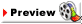This animation has audio.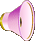Category : Math Integer Type : Animation with sound Animation Type : Regular Total animation length: 47 minutes The animation covers: What are these new negative numbers? What are positive numbers? What are the uses of negative numbers? Representing integers on a number line What do we get from the number line? Why do bigger negative numbers are said to be smaller? History on number line Opposite integer or additive inverse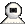Snapshots
 Watch combined previewWatch various movie clips related to integers. These are 1) Absolute value of an integer 2) Arithmetic operations of Integers 3) BODMAS Rule 4) Introduction to Integers 5) Number line - Representing History 6) Introduction to Natural and Whole Numbers 7) Representing Integers on Number line 8) Quiz on Integers. Click on the above thumbnails to go to the movie.A real life example clearly explains the concept of a number line.This is like an animated math tutorial but with entertainment and stories to make learning number line fun. We have provided many examples to clarify doubts in number line.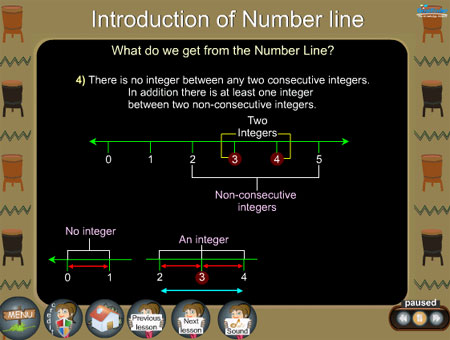Rules of number line are clearly explained.Details of the animation/ movie /software

Introduction to Integers with real life examples is the basis of the animation.

Introduction to integers and positive and negative numbers are supported with more examples. Positive integers means things associated with increasing, ascending, gain etc while decreasing, descending, loss etc denote negative integers.

Another example introduces representing integers on a number line, rules are needed for understanding how to write integers on a number line, for example: An integer is always larger than one on its left.

Then the concept of additive inverse is brought out through number line and real life examples. Example: +31 and -31.

The animation covers the introduction to integers using real life examples.Related Products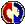Buyers Feedback:Give your comments